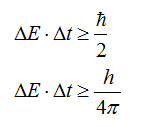# A pion and a proton can briefly join together to form a delta particle. A measurement of the energy of the system shows a peak at 1236 MeV, corresponding to the rest energy of the delta particle, with an experimental spread of 120 MeV. What is the lifetime of the delta particle?

Question
6 views

A pion and a proton can briefly join together to form a delta particle. A measurement of the energy of the system shows a peak at 1236 MeV, corresponding to the rest energy of the delta particle, with an experimental spread of 120 MeV. What is the lifetime of the delta particle?

check_circle

Step 1

Let the experimental spread of the energy corresponding to the rest energy of Δ  (delta)particle be denoted as ΔE.

Let the lifetime of the Δ (delta) particle be denoted as Δt.

Use the Heisenberg’s uncertainty principle to calculate the lifetime of the delta particle as,...

### Want to see the full answer?

See Solution

#### Want to see this answer and more?

Solutions are written by subject experts who are available 24/7. Questions are typically answered within 1 hour.*

See Solution
*Response times may vary by subject and question.
Tagged in